# Java SimpleITK Slice extraction and display with JavaFX

Hi,

i am trying to get a slice from any axis like axial, sagital or coronal from dicom volume,
i already made it with python, but due to python limitations i migrate to java.

this is how i load the volume:

``````public static org.itk.simple.Image loadLocalFolder (Stage stage) throws Exception {
}
``````

this is how i get the slice:

``````public static Image axisSlice (Image volume, long[] sliceSize, int[] sliceIndex) {
VectorUInt32 vectorSize = new VectorUInt32(sliceSize);
VectorInt32 vectorIndex = new VectorInt32(sliceIndex);
return SimpleITK.extract(volume, vectorSize, vectorIndex);
}
``````

with the result of axisSlice, i’m trying to display it in my application with javaFX.
So i made a function that transform the extractedImage in a byte array:

``````public static byte[] volumeToByteArray (Image volume) {
ByteBuffer imageDataBuffer = volume.getBufferAsByteBuffer();
byte[] imageByteArray = new byte[imageDataBuffer.remaining()];
imageDataBuffer.get(imageByteArray);
return imageByteArray;
}
``````

from here things starts getting a little weird.
example: if the extracted image size is 512x512, im expeting volumeToByteArray return a 262.144 byte array lenght. But it is returning twice as much, so the returned byte array has 524.288 lenght.

A variable of type byte can have a value between -128 and 127, so it has a range of 256 possible values.
As dicom files only have black and white colors, my understanding is that each byte should represent an RGB grayscale pixel. So RGB(0, 0, 0) is totally black, and RGB(255, 255, 255) is totally white.

You can see this by typing “colorpicker” in google: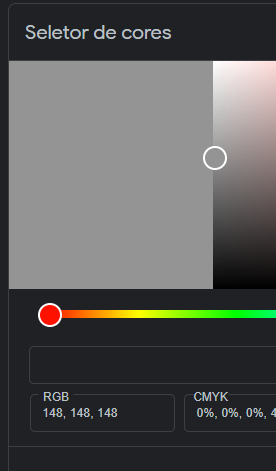i understand that this is not a javafx community, but trying to create the images from byte array data result in unsatisfactory results, maybe meaning that the byte array data isnt correct. I can only open a question on stackoverflow now.

The fact that converting the image to a byte array is returning a vector with twice the expected size can fit here, can anyone help me?

java created image from a dicom folder, from axisSlice(volume, {512, 512, 0}, {0, 0, 10}):

python created image from same dicom folder, from axisSlice(volume, {512, 512, 0}, {0, 0, 10}):

java image generator code:

``````public static WritableImage volumeToImage (Image volume) {
byte[] imageByteArray = Slicer.volumeToByteArray(volume);
int imageHeight = volume.getSize().get(0).intValue();
int imageWidth = volume.getSize().get(1).intValue();
WritableImage writableImage = new WritableImage(imageWidth, imageHeight);
PixelWriter pixelWriter = writableImage.getPixelWriter();
int line;
int column;
int maxIndex = imageHeight*imageWidth;
for (int index = 0; index < maxIndex; index++) {
line = (index/imageWidth);
column = index - line*imageWidth;
int grayIntensity = imageByteArray[index] + 128;
pixelWriter.setColor(line, column, javafx.scene.paint.Color.rgb(grayIntensity, grayIntensity, grayIntensity));
}
return writableImage;
}

Image extractedImage = Slicer.axisSlice(volume.getVolume(), new long[]{512, 512, 0}, new int[]{0, 0, 10});
WritableImage writableImage = Slicer.volumeToImage(volume.getVolume());
Viewport.imageView.setImage(writableImage);

``````

Hello @Salu_Ramos,

Your assumption with respect to the image content is incorrect.

MRI and CT images have a high dynamic intensity range, intensity values with a range much larger than [0,255]. In your case the data is represented by 16bits per pixel, so two bytes, which matches the size of the returned byte array. To check the human readable pixel type use the image’s `GetPixelIDTypeAsString` method.

Displaying high dynamic range images in the medical domain is usually done by mapping them to the range [0,255] via a window level operation, IntensityWindowingImageFilter before display. For CT there are typical W/L values.

3 Likes

Hi @zivy ,

this help me a lot, i changed my code:

``````public static WritableImage volumeToImage (Image volume) throws InterruptedException {
byte[] imageByteArray = Slicer.volumeToByteArray(volume);
int imageHeight = volume.getSize().get(0).intValue();
int imageWidth = volume.getSize().get(1).intValue();
WritableImage writableImage = new WritableImage(imageWidth, imageHeight);
PixelWriter pixelWriter = writableImage.getPixelWriter();
int line;
int column;
int pixelBytes = (int) volume.getSizeOfPixelComponent();

for (int index = 0; index < imageByteArray.length; ) {
long grayIntensity = 0;
for (int pixel = 0; pixel < pixelBytes; pixel++) {
int value = imageByteArray[index + pixel] + 128;
int weight = (int) Math.pow(256, pixelBytes - pixel - 1);
grayIntensity += (long) value * weight;
}
int mappedGrayIntensity = (int) (grayIntensity/Math.pow(256, pixelBytes - 1));
int actualElement = (int) Math.floor((double) index /pixelBytes);
line = (actualElement/imageWidth);
column = actualElement - line*imageWidth;
pixelWriter.setColor(line, column, javafx.scene.paint.Color.rgb(mappedGrayIntensity, mappedGrayIntensity, mappedGrayIntensity));
index += pixelBytes;
}
return writableImage;
}
``````

Now things are flowing better, but the result is still not ideal

Basically, i’m reading the bytes 2 by 2 in this case, applying a weight of 256 por first byte, and weight 1 for second byte, adding it all up and mapping to [0, 256] scale. But as you see the image has too much gray, i dont think i’m doing the right operation.

Hello @Salu_Ramos,

What you are seeing is overflow due to an error in your computation. I would recommend that you use SimpleITK to get a valid byte buffer in [0,255] as follows. Take the SimpleITK 2D image, use the `IntensityWindowingImageFilter` to map the values to [0,255] then `CastImageFilter` to make the result a UInt8 image. Finally, get the bytearray from that image.

1 Like

thanks @zivy!

if i understood it straight, i must apply the filter before generating the byte array…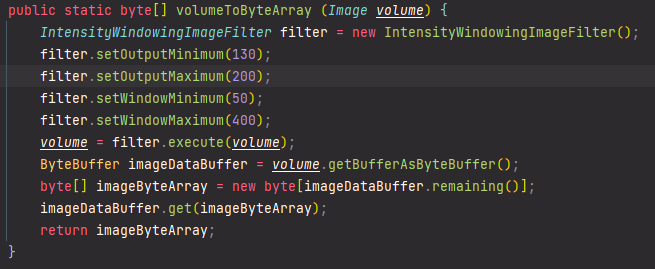and the values ​​that I should put inside SetWindow and SetOutput should be respectively the W and L of that link you sent me, according to the type of image, is that alright?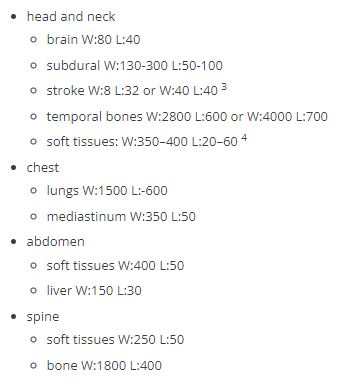my result:

Hello @Salu_Ramos,

Yes, apply the `IntensityWindowingImageFilter` before getting the byte array, also do the Cast, so that the byte array is as you expect it to be (otherwise its size will not match what you expect). The intensity change only changes the values in the pixels, not how they are represented (2bytes per pixel).

With respect to the values to use for window/leveling, the ones I shared are for CT. You are working with MR, so different values. The appropriate values are up to your users. You may want to allow them to set them manually via the GUI.

1 Like

thanks for your reply again @zivy , i managed to understand the W and L properties.

example of abdomen->soft issues: W:400 L:50
gray represents density.
-1000 is black, represents air.
+1000 is white, represents metal.
In this case center is L = 50, and range is [L-W/2 ; L+W/2], so range is [-150;250]

1 Like

Hello @zivy, i’m coming back after some time, not sure if this filter is being applied correctly.

``````public static Image brightnessAndContrastFilter (Image volume, double wl, double ww) {
IntensityWindowingImageFilter filter = new IntensityWindowingImageFilter();
filter.setOutputMinimum(0);
filter.setOutputMaximum(255);
filter.setWindowMinimum(wl - (ww/2));
filter.setWindowMaximum(wl + (ww/2));
return filter.execute(volume);
}
``````

i have this presets in my software: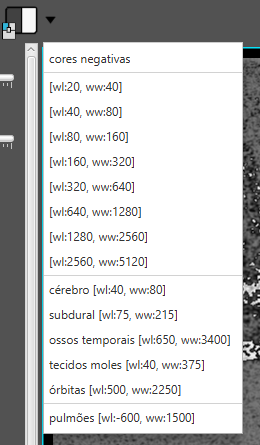my output for [wl:20 ,ww:40]:

radiant dicom viewer output for [wl:20 ,ww:40]: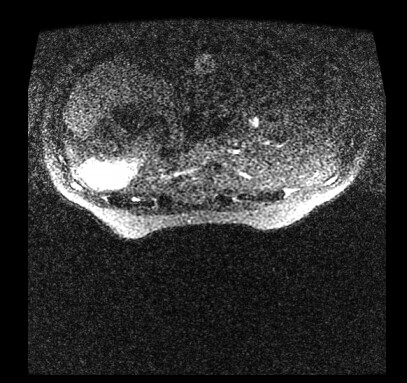Hello @Salu_Ramos,

Your code looks reasonable to me, so not sure what the radiant viewer is doing differently.

To quickly see if W/L conversion to min/max values make sense, open your image using ITK-SNAP, then Tools->Image Contrast → Contrast Adjustment. The GUI will let you play with the WL values and you will see the corresponding min/max values that you need to use, so you can confirm that your code behaves correctly.

1 Like

Hi @zivy, very cool, i’m understanding a little more whats going on with the help of itk-snap.

changing the subject.
my image reconstruction function are having some problems with specific dicom files. One in special is am exam that each pixel has red, green and blue. I discovered it because even after applying castImageFilter the imageByteArray.lenght is 3 times bigger than extractedImage.getNumberOfPixels()

expected output:

this special file throws this error when trying to run IntensityWindowingImageFilter:

``````Exception thrown in SimpleITK IntensityWindowingImageFilter_execute: D:\a\1\sitk\Code\Common\include\sitkMemberFunctionFactory.hxx:158:
sitk::ERROR: Pixel type: vector of 8-bit signed integer is not supported in 2D by class itk::simple::IntensityWindowingImageFilter.
``````

castImageFilter function as you said earlier:

``````public static Image castImageFilter (Image extractedImage) {
CastImageFilter filter = new CastImageFilter();
filter.setOutputPixelType(PixelIDValueEnum.sitkVectorUInt8);
return filter.execute(extractedImage);
}
``````

my actual image reconstruction function:

``````public static WritableImage volumeToWritableImageWithCPU(Image extractedImage, boolean negativeColor) {
extractedImage = Filters.castImageFilter(extractedImage);
byte[] imageByteArray = volumeToByteArray(extractedImage);
int imageHeight = extractedImage.getSize().get(1).intValue();
int imageWidth = extractedImage.getSize().get(0).intValue();
WritableImage writableImage = new WritableImage(imageWidth, imageHeight);
PixelWriter pixelWriter = writableImage.getPixelWriter();
int column;
int line;
int pixelBytes = (int) extractedImage.getNumberOfComponentsPerPixel();
int rgbComponents = imageByteArray.length/extractedImage.getNumberOfPixels().intValue();
if (rgbComponents == 1) {
for (int index = 0; index < imageByteArray.length; ) {
long grayIntensity = 0;
for (int pixel = 0; pixel < pixelBytes; pixel++) {
int value = imageByteArray[index + pixel] + 128;
double weight = (int) Math.pow(256, pixelBytes - pixel - 1);
grayIntensity += value * weight;
}
int mappedGrayIntensity = (int) (grayIntensity/Math.pow(256, pixelBytes - 1));
if (negativeColor) {
mappedGrayIntensity = 255 - mappedGrayIntensity;
}
int actualElement = (int) Math.floor((double) index /pixelBytes);
column = (actualElement/imageHeight);
line = actualElement - column*imageHeight;
pixelWriter.setColor(column, line, javafx.scene.paint.Color.rgb(mappedGrayIntensity, mappedGrayIntensity, mappedGrayIntensity));
index += pixelBytes;
}
} else if (rgbComponents == 3) {
for (int index = 0; index < imageByteArray.length; ) {
int actualElement = (int) Math.floor((double) index / rgbComponents);
column = (actualElement/imageHeight);
line = actualElement - column*imageHeight;
pixelWriter.setColor(column, line, javafx.scene.paint.Color.rgb(imageByteArray[index]+128, imageByteArray[index + 1]+128, imageByteArray[index + 2]+128));
index += rgbComponents;
}
}
return writableImage;
}
``````

my output:

the others file results are ok.

Hello @Salu_Ramos ,

The bug isn’t immediately obvious to me. Possibly check what are the three pixel values in a specific index. I don’t remember if the byte array is ordered `r0, g0, b0, r1, g1, b1...` or `r0, r1...g0, g1..., b0,b1...`.

1 Like

The image your window/leveling produced looks like the pixel values are overflowing. If you compare the brightest regions of the radiant viewer with your image, in yours those brightest pixel are grey. That suggests overflowing to me. Maybe the input images are UInt8 type. Try converting them to Float32 before doing any math.

1 Like

@dchen, yes the input images are Uint8! when i try to convert to VectorFloat32 my byte array gets very big, i dont know what to do with this, so cant get any output.
When using castImageFilter to VectorFloat32 the array lenght is 11.615.184, while VectorUInt8 array lenght is 2.903.796

@zivy, i have the same doubt.
additional information i get from the dicom file: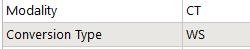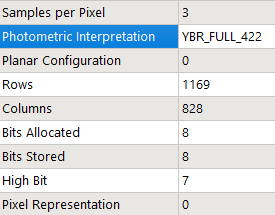after some time trying to understand what was going on, I noticed that the rows and columns were swappedthis took a while to cross my mind as the other files looked correct

my actual output RGB: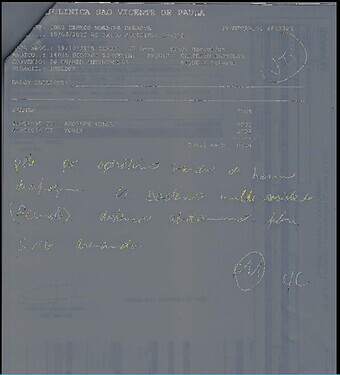That’s not that big in the grand scheme of things. But you could try converting your RGB images to gray scale, since you don’t really seem to have color data.

1 Like

@dchen, yeah i think you are right, that strategy is getting me nowhere.

but the image has color data.
this is the expected output: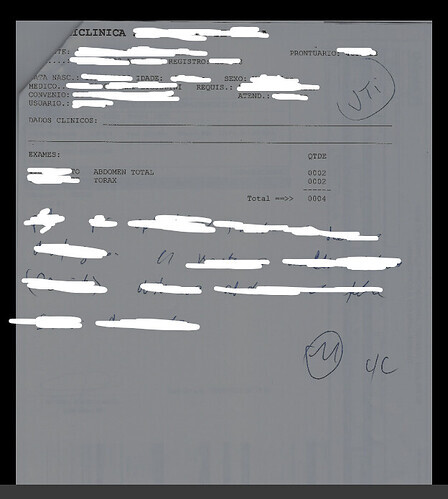my actual output are not right, the writing should be blue but instead there is just image noise.

i already converted to grayscale as following tutorial:
https://www.mvtec.com/doc/halcon/12/en/rgb1_to_gray.html

my actual function:

``````public static WritableImage volumeToWritableImageWithCPU(Image extractedImage, boolean negativeColor) {
if (extractedImage.getPixelID() != PixelIDValueEnum.sitkVectorUInt8) {
extractedImage = Filters.convertImageToVectorUInt8(extractedImage);
}
byte[] imageByteArray = volumeToByteArray(extractedImage);
int imageHeight = extractedImage.getSize().get(0).intValue();
int imageWidth = extractedImage.getSize().get(1).intValue();
WritableImage writableImage = new WritableImage(imageHeight, imageWidth);
PixelWriter pixelWriter = writableImage.getPixelWriter();
int column;
int line;
int pixelBytes = (int) extractedImage.getNumberOfComponentsPerPixel();
int rgbComponents = imageByteArray.length/extractedImage.getNumberOfPixels().intValue();
if (rgbComponents == 1) {
for (int index = 0; index < imageByteArray.length; ) {
long grayIntensity = 0;
for (int pixel = 0; pixel < pixelBytes; pixel++) {
int value = imageByteArray[index + pixel] + 128;
double weight = (int) Math.pow(256, pixelBytes - pixel - 1);
grayIntensity += value * weight;
}
int mappedGrayIntensity = (int) (grayIntensity/Math.pow(256, pixelBytes - 1));
if (negativeColor) {
mappedGrayIntensity = 255 - mappedGrayIntensity;
}
int actualElement = (int) Math.floor((double) index /pixelBytes);
column = (actualElement/imageHeight);
line = actualElement - column*imageHeight;
pixelWriter.setColor(line, column, javafx.scene.paint.Color.grayRgb(mappedGrayIntensity));
index += pixelBytes;
}
} else if (rgbComponents == 3) {
for (int index = 0; index < imageByteArray.length; ) {
int actualElement = (int) Math.floor((double) index/rgbComponents);
column = (actualElement/imageHeight);
line = actualElement - column*imageHeight;
int red = (imageByteArray[index] + 128);
int green = (imageByteArray[index+1] + 128);
int blue = (imageByteArray[index+2] + 128);
//                int gray = (int) (0.299 * (imageByteArray[index]+128) + 0.587 * (imageByteArray[index+1]+128) + 0.114 * (imageByteArray[index+2]+128));
pixelWriter.setColor(line, column, javafx.scene.paint.Color.rgb(red, green, blue));
index += rgbComponents;
}
}
return writableImage;
}
``````

Turns out the problem was on lines such as

`int color = (imageByteArray[index] + 128);`

The correct way to parse the color bytes is

`int color = (imageByteArray[index] & 0xFF);`

1 Like

I would suggest trying to do image operations in SimpleITK before converting your image into a WritableImage. Using the mathematical filters in SimpleITK such as AddImageFilter and PowImageFilter should have much better performance than looping over pixels Java.

1 Like

this little change really fixed everything.

``````public static WritableImage viewportRenderImageWithCPU(Image extractedImage, boolean negativeColor) {
if (extractedImage.getPixelID() != PixelIDValueEnum.sitkVectorUInt8) {
extractedImage = Filters.convertImageToVectorUInt8(extractedImage);
}
byte[] imageByteArray = volumeToByteArray(extractedImage);
int imageWidth = extractedImage.getSize().get(0).intValue();
int imageHeight = extractedImage.getSize().get(1).intValue();
WritableImage writableImage = new WritableImage(imageWidth, imageHeight);
PixelWriter pixelWriter = writableImage.getPixelWriter();
int column;
int line;
int rgbComponents = imageByteArray.length/extractedImage.getNumberOfPixels().intValue();
if (rgbComponents == 1) {
for (int index = 0; index < imageByteArray.length; index++) {
int grayIntensity = imageByteArray[index] & 0xFF;
if (negativeColor) {
grayIntensity = 255 - grayIntensity;
}
column = (index/imageWidth);
line = index - column*imageWidth;
pixelWriter.setColor(line, column, javafx.scene.paint.Color.grayRgb(grayIntensity));
}
} else if (rgbComponents == 3) {
for (int index = 0; index < imageByteArray.length; index += rgbComponents) {
int actualElement = (int) Math.floor((double) index/rgbComponents);
column = (actualElement/imageWidth);
line = actualElement - column*imageWidth;
int red = (imageByteArray[index] & 0xFF);
int green = (imageByteArray[index+1] & 0xFF);
int blue = (imageByteArray[index+2] & 0xFF);
//                int gray = (int) (0.299 * (imageByteArray[index]+128) + 0.587 * (imageByteArray[index+1]+128) + 0.114 * (imageByteArray[index+2]+128));
pixelWriter.setColor(line, column, javafx.scene.paint.Color.rgb(red, green, blue));
}
}
return writableImage;
}
``````